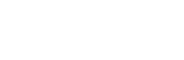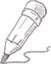#CDA持证人阿涛哥

2023-09-14   阅读量: 1146

## CDA模拟题学习笔记之某地区成年女子的血压(以mmHa计) 服从N(110，144)，求该地区成年女性血压在100至120的可能性多大?A 0.676

B 0.5

C 0.5934

D 0.5768

Z = (X - μ) / σ

Z1 = (100 - 110) / 12 = -0.8333

Z2 = (120 - 110) / 12 = 0.8333

P1 = φ(-0.8333) = 1 - φ(0.8333) = 1 - 0.7975 = 0.2025

P2 = φ(0.8333) = 0.7975

P2 - P1 = 0.7975 - 0.2025 = 0.595

0.0000 1 0 关注作者 收藏

## 评论(0)